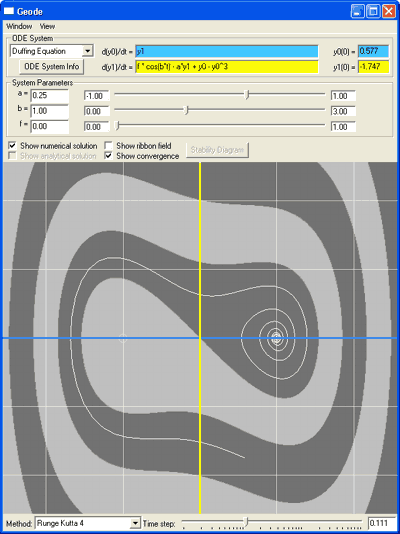# Geode

 Geode is a visualization tool for understanding ordinary differential equations and the algorithms that are used to solve them on computers.  With it, you can: type in any system of two ODEs (including non-autonomous systems) or select one of several interesting preconfigured systems numerically integrate an ODE system using one of several standard ODE solvers, including 4th-order Runge-Kutta and the backward Euler method. for linear ODEs, compare the numerical result to the analytical solution explore the stability properties of various ODE solvers in an interactive visualization of their imaginary plane stability regions visualize the convergence behavior of non-linear ODE systems under various solvers with a convergence-point-based coloring of the plane save and load ODE systems and settings We expect that Geode will be useful for students of numerical analysis who would like to gain a better understanding of the behavior of systems of ordinary differential equations and their solvers. Questions?  Bugs? Jeff Klingner (klingner at graphics.stanford.edu) or Dave Akers (dakers at graphics.stanford.edu).